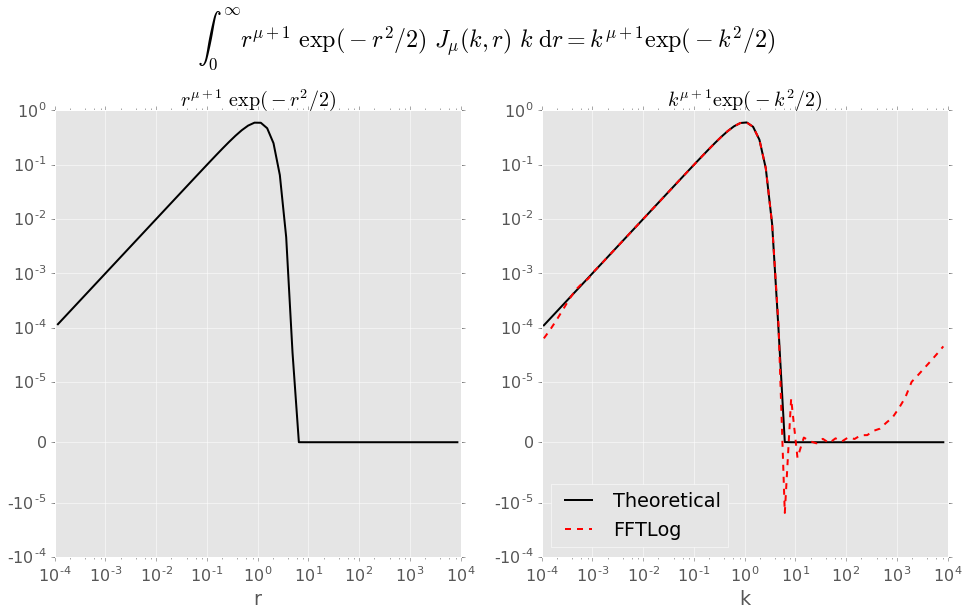# fftlog

#### 01 October 2016

If you are looking for a fast Fourier transform (FFT) that works flawlessly over many orders of magnitudes you might want to search for a logarithmic FFT. And if you do search for a logarithmic FFT, you most likely will end up at Andrew Hamilton’s website for FFTLog (published in Appendix B of Hamilton, 2000). Because, as it states on the website, “FFTLog can be regarded as a natural analogue to the standard Fast Fourier Transform … [for] a logarithmically spaced periodic sequence“.

FFTLog is written in Fortran. And if you followed this blog than you might be aware that I do most of my scripting in Python. I used FFTLog a while ago during my Ph.D. Last week I needed for a project again a logarithmically spaced FFT routine, so I dug out my old files. I improved the wrapping slightly over my old version and moved them from Python 2 to Python 3. For the wrapping I use the great f2py-module, baked right into numpy these days.

In order to be faster up and running next time I need FFTLog in Python, and as it might prove useful for others, I put the simply fftlog named module up on GitHub: fftlog on GitHub, published under the Public Domain Dedication (CC0 1.0).

Update 05 December 2016: I rewrote FFTLog completely in Python, using scipy.fftpack and scipy.special.loggamma. The module pyfftlog is slightly slower than fftlog, but easier to implement, as it is just one Python-file, and there is nothing to install or compile. You can get pyfftlog on GitHub.

## Notebook fftlogtest.ipynb

For testing purposes I translated the test-file fftlogtest.f into a Jupyter notebook, which can be found in the projects root directory. The following are cells 4-19 of that notebook to illustrate the usage of fftlog (direct link to the complete notebook).

In :
import fftlog
import numpy as np
import matplotlib as mpl
import matplotlib.pyplot as plt

%matplotlib inline
plt.style.use('ggplot')
mpl.rcParams.update({'font.size': 16})


### Define the parameters you wish to use¶

The presets are the Reasonable choices of parameters from fftlogtest.f.

In :
# Range of periodic interval
logrmin = -4
logrmax = 4

# Number of points (Max 4096)
n = 64

# Order mu of Bessel function
mu = 0

# Bias exponent: q = 0 is unbiased
q = 0

# Sensible approximate choice of k_c r_c
kr = 1

# Tell fhti to change kr to low-ringing value
# WARNING: kropt = 3 will fail, as interaction is not supported
kropt = 1

# Forward transform (changed from dir to tdir, as dir is a python fct)
tdir = 1

In :
# Central point log10(r_c) of periodic interval
logrc = (logrmin + logrmax)/2

print('Central point of periodic interval at log10(r_c) = ', logrc)

# Central index (1/2 integral if n is even)
nc = (n + 1)/2.0

# Log-spacing of points
dlogr = (logrmax - logrmin)/n
dlnr = dlogr*np.log(10.0)

Central point of periodic interval at log10(r_c) =  0.0


### Calculate input function: $r^{\mu+1}\exp\left(-\frac{r^2}{2}\right)$¶

In :
r = 10**(logrc + (np.arange(1, n+1) - nc)*dlogr)
ar = r**(mu + 1)*np.exp(-r**2/2.0)


### Initialize FFTLog transform - note fhti resets kr¶

In :
kr, wsave, ok = fftlog.fhti(n, mu, dlnr, q, kr, kropt)
print('fftlog.fhti: ok =', bool(ok), '; New kr = ', kr)

fftlog.fhti: ok = True ; New kr =  0.9535389675791912


### Call fftlog.fht (or fftlog.fftl)¶

In :
logkc = np.log10(kr) - logrc
print('Central point in k-space at log10(k_c) = ', logkc)

# rk = r_c/k_c
rk = 10**(logrc - logkc)

# Transform
#ak = fftlog.fftl(ar.copy(), wsave, rk, tdir)
ak = fftlog.fht(ar.copy(), wsave, tdir)

Central point in k-space at log10(k_c) =  -0.0206615542605


### Calculate Output function: $k^{\mu+1}\exp\left(-\frac{k^2}{2}\right)$¶

In :
k = 10**(logkc + (np.arange(1, n+1) - nc)*dlogr)
theo = k**(mu + 1)*np.exp(-k**2/2.0)


### Plot result¶

In :
plt.figure(figsize=(16,8))

# Transformed result
ax2 = plt.subplot(1, 2, 2)
plt.plot(k, theo, 'k', lw=2, label='Theoretical')
plt.plot(k, ak, 'r--', lw=2, label='FFTLog')
plt.xlabel('k')
plt.title(r'$k^{\mu+1} \exp(-k^2/2)$', fontsize=20)
plt.legend(loc='best')
plt.xscale('log')
plt.yscale('symlog', basey=10, linthreshy=1e-5)
ax2ylim = plt.ylim()

# Input
ax1 = plt.subplot(1, 2, 1)
plt.plot(r, ar, 'k', lw=2)
plt.xlabel('r')
plt.title(r'$r^{\mu+1}\ \exp(-r^2/2)$', fontsize=20)
plt.xscale('log')
plt.yscale('symlog', basey=10, linthreshy=1e-5)
plt.ylim(ax2ylim)

# Main title
plt.suptitle(r'$\int_0^\infty r^{\mu+1}\ \exp(-r^2/2)\ J_\mu(k,r)\ k\ {\rm d}r = k^{\mu+1} \exp(-k^2/2)$',
fontsize=24, y=1.08)
plt.show()## Installation

To install fftlog into your python distribution, execute

$python setup.py install  To just create the module that you can import locally, execute $ f2py -c fftlog.pyf src/*


## Notes

1. kropt = 3 (interactive adjusting) is not possible with fftlog
2. wsave-dimension is set to 3.5*n+19, the biggest of the four minimum sizes described in fftlog.f.

## Creation

I did very little for the whole thing, the power of f2py did most of the work.

The src-directory contains the original Fortran files as downloaded from casa.colorado.edu/~ajsh/FFTLog. The only change I made was to recode the coding of fftlog.f, as f2py struggled with a few characters in the description part:

recode latin1..UTF-8 fftlog.f


Thereafter I used f2py to produce the pyf-instructions with the following command, generating only hooks for the functions fhti, fttl, fht, and fhtq:

f2py src/* -m fftlog -h fftlog.pyf only: fhti fftl fht fhtq :


Lastly I amended the pyf-instructions, mainly with some intent and optional statements as well as the corresponding default values.